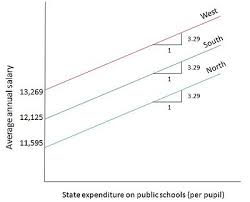### K-means and Cost Modeling

For k-means there are a lot of use cases. One of these use cases is the ability to do non-linear cost modeling. In this podcast episode, we explore the ability to do cost modeling. K-means is not able to give you a predicted cost for a new product directly, unless you want to build your model so that it just assigns the new product to a cluster and returns the average cost for that cluster. That would be a very boring model.

One way to deal with this is to set a regression model on top of your clustering. The clusters from the initial pass are then fed into the regression for cost, and thus you get a unique cost. This clustering helps to break the linearity of OLS.

There are a couple of ways to do this. The first is to assume that all of your products have the same cost structure, and simply have different means. So to solve this problem, k-means will give you some clustering and allow you to shift the mean of the cluster. In this way you can predict what your cost will be for a new product in that cluster. In essence, you are using cluster membership as a dummy variable.same slopes, different intercepts

The idea is really basic. It will introduce the non-linear aspect to the cost modeling that you are looking for, but it really doesn’t get you to where you want to go. I mean the assumption that you have the same cost structure for every product, regardless of what type of product, cluster membership, etc. is a very restrictive assumption.

There is another way that you can treat this data. What I am thinking is that you could use the clusters to not only shift the mean of the cost model, but you can also shift the slope. In that way you can talk about the cost model for the cluster. In the episode, I talk about one way to do that is to create a separate model for each cluster. I don’t like that way of thinking. I want a model that is simpler than that. The way that I solve that problem is by multiplying my cluster dummy to every variable. That gives me a really nice alternative to run a single OLS model to generate different cost models for each cluster.different means and different slopes

I like this approach because it simplifies life. Plus it has an added benefit that I barely touched on in the episode itself. You get to do fuzzy cost modeling. So the idea is that you train a k-means model on a subset of the data. From this subset you now have the information that you need to build a classification algorithm. What are you trying to predict? Cluster membership. Your clustering algorithm will spit out a probability at you for each cluster. Now, when you get a new product, you have an algorithm which will assign it to a cluster. But even better, you have the probability that it belongs to a cluster.

Now instead of multiplying each variable by the dummy for the cluster that it is most likely to belong in, multiply each variable by the cluster probabilities and feed that into your regression. If you do that, then you will have a very non-linear, very product-specific model for costs.

This set up for cost modeling is extremely flexible. It is also a really cool project for people starting out in data science. The reason is that it gives you exposure in a real world setting to all 3 major algorithm types, clustering, regression, and classification. Kind of cool, right?